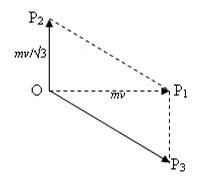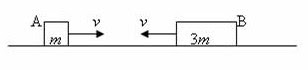Pages

Life is like riding a bicycle.  To keep your balance you must keep moving.”
Albert Einstein

Sunday, October 23, 2011

AP Physics B & C - Multiple Choice Practice Questions on Work, Energy and Power

“The best thinking has been done in solitude. The worst has been done in turmoil.”

– Thomas A. Edison

Today we will discuss a few multiple choice practice questions involving work, energy and power. Questions in this section were discussed earlier on this site. You can access them by clicking on the label ‘work energy power’ or by trying a search for the required word using the search box provided on this page.

(1) A sphere A of mass m moves with speed v and kinetic energy E along the positive x-direction and collides inelastically with another identical sphere B at rest. After the collision sphere A moves with kinetic energy E/3 along the positive y-direction. What is the speed of sphere B after the collision?

(a) v/2

(b) v(√3)/2

(c) v(√5)/2

(d) 2v/√3

(e) 4v/√3

We have E = ½ mv2

Since the kinetic energy is directly proportional to the square of speed, the speed of A after the collision is v/√3.

Momentum is conserved in all collisions (elastic as well as inelastic). The momentum of sphere A before the collision is mv and is directed along the positive x-direction. This is the total momentum of the system since the initial momentum of sphere B is zero.The momentum mv is represented by the vector OP1 in the adjoining figure. The momentum of sphere A after the collision is mv/√3 and is directed along the positive y-direction. This is represented by the vector OP2. The momentum of sphere B after the collision must be given by the vector OP3 since the parallelogram OP2P1P3 is drawn such that its diagonal OP1 represents the final momentum, mv of the system

Evidently OP32 = OP12 + │OP22

Or, OP32 = (mv)2 + (mv/√3)2

But OP3│ = mvf where vf is the speed of sphere B after the collision.

Thus we have

(mvf)2 = (mv)2 + (mv/√3)2

This gives vf = √(v2 + v2/3) = 2v/√3

(2) A particle moves in a plane under the action of a force of constant magnitude. If the direction of the force is always at right angles to the direction of motion of the particle, it follows that

(a) the momentum of the particle is constant

(b) the velocity of the particle is constant

(c) the kinetic energy of the particle is constant

(d) the acceleration of the particle is constant

(e) the particle moves along a straight line with increasing speed

A force of constant magnitude acting always at right angles to to the direction of motion of the particle will supply centripetal force required for uniform circular motion. In uniform circular motion the speed of the particle is constant.

[The velocity is not constant since the direction changes continuously. The momentum and the acceleration also are not constant for the same reason].

Since the speed is constant, the kinetic energy of the particle is constant [Option (c)].

(3) A ball of mass 0.5 kg is thrown vertically downwards from the edge of a building of height 65 m. The initial speed of the ball is 20 ms–1. If the ball strikes the ground with a speed of 40 m/s, the energy lost by the ball because of air resistance is (gravitational acceleration, g = 10 ms–2)

(a) 10 J

(b) 25 J

(c) 30 J

(d) 40 J

(e) 45 J

The initial energy of the ball is Ei = mgH + ½ mvi 2

The first term is the gravitational potential energy of the ball of mass m at height H and the second term is the initial kinetic energy of the ball having initial speed vi. Substituting proper values, we have,

Ei = 0.5×10×65 + ½ ×0.5×202 = 325 + 100 = 425 J.

The final energy of the ball (at the ground level) having velocity vf is Ef = ½ mvf2

Substituting proper values, we have,

Ef = ½×0.5×402 = 400 J.

Therefore, the energy lost by the ball because of air resistance is Ei Ef = 425 J 400 J = 25 J.(4) Two blocks A and B of masses m and 3m respectively (Fig.) travel in opposite directions with the same speed v along a smooth surface. They collide head-on and stick together. Mechanical energy lost during the collision is

(a) ½ mv2

(b) mv2

(c) (3/2) mv2

(d) 2 mv2

(e) 3 mv2

Total momentum of the system before collision is 3mvmv = 2 mv

[We have taken the leftward momentum as positive and that’s why the total momentum is positive. If you take the leftward momentum as negative, the total momentum will be negative. But this won’t affect your final answer and the energy lost during the collision will be positive].

Since the momentum of the system is conserved, the total momentum after the collision will be 2 mv itself. If the common velocity of A and B (which stick together) after collision is vf, we have,

(m + 3m)vf = 2 mv

Therefore vf = v/2

The initial kinetic energy of the system is ½ mv2 + ½ (3m)v2 = 2 mv2

Final kinetic energy of the system is ½ (4m)vf2 = ½ (4m)(v/2)2 = ½ mv2

Therefore, the loss of kinetic energy during the collision = 2 mv2 ½ mv2 = (3/2) mv2

(5) An electric motor creates a tension of 1000 N in a hoist cable and reels it at the rate of 2.5 ms–1. The power output of the motor is

(a) 250W

(b) 400 W

(c) 2.5 kW

(d) 2500 kW

(e) 400 kW

Since the point of application of the force of 1000 N moves through 2.5 m per second, the work done per second (which is the power output) is 1000×2.5 watt = 2500 watt = 2.5 kW